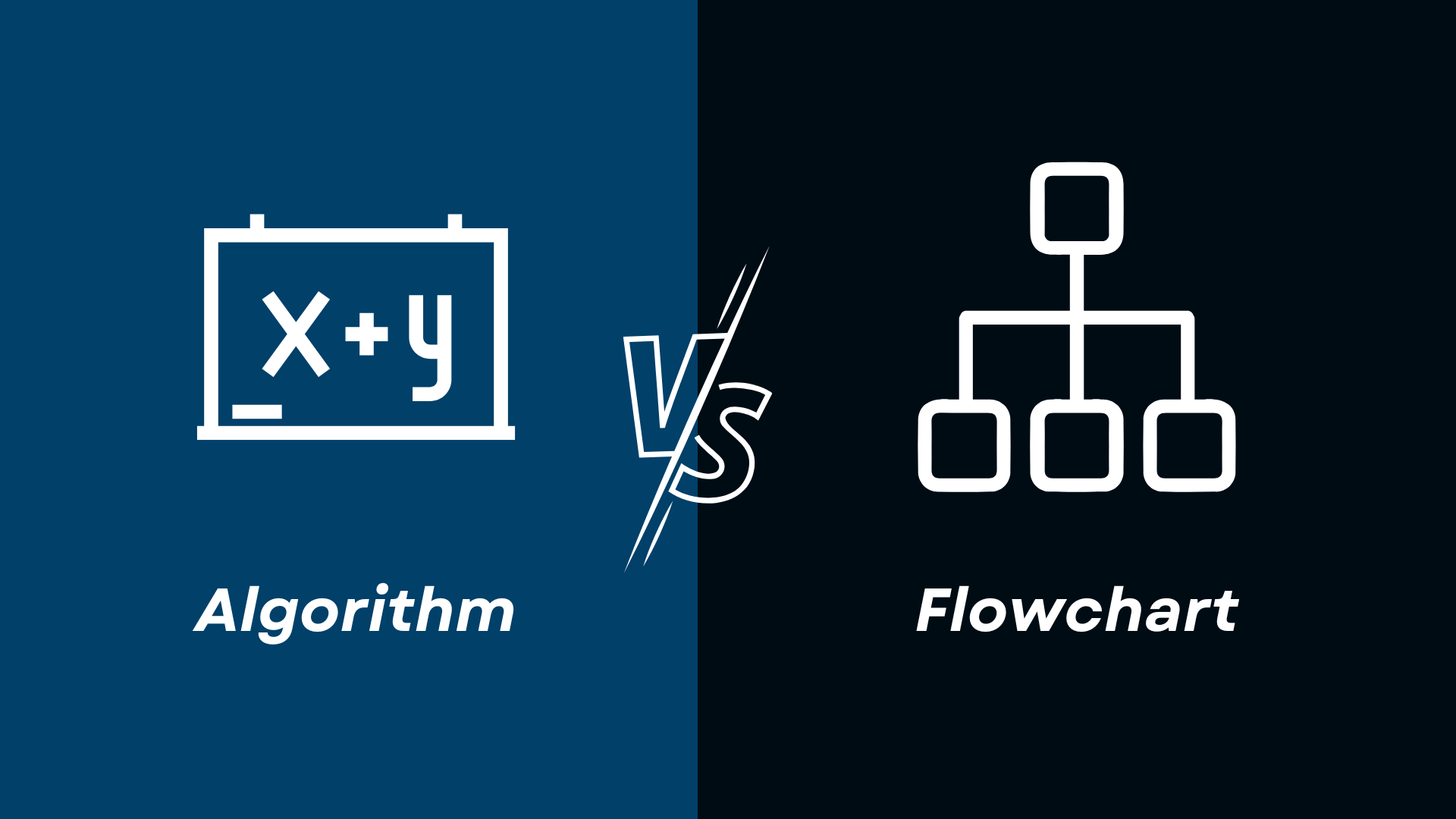# Algorithm and Flowchart: Differences Explained## What is an Algorithm?

Algorithms and flowcharts are used for the representation of a program in a different manner, especially in computer programming. An algorithm is a step-by-step procedure, which has to be followed when an input is given to obtain the desired output or result. It is generally represented in form of steps during the execution. Algorithms give a vague idea of logic to be implemented to get the desired output from the given input.

### Example:

Let's take an example of a simple program for better understanding of algorithms in computer programming. Below is the algorithm to be followed to print the sum, average of given 5 numbers.

### Steps to be followed:

1. Take 5 numbers as input from the user. Let them be A1, A2, A3, A4, A5.
2. Now, sum the values of (A1+A2+A3+A4+A5) and store them in a variable called sum.
3. Average of five numbers is sum/5 which is stored in variable avg.
4. Return the values stored in sum, avg as output.

## What is a Flowchart?

On the other hand, a flowchart is a graphical representation of the steps to be followed of a program takes to obtain the desired output. Flowchart uses many geometric shapes to indicate different steps. Each geometric shape represent one different step. For example, a diamond shape indicates an execution of if/else statement. An oval/ round rectangle represents the start and end of the program. A rectangle shape indicates the block of code which consists of numbers manipulation or printing statements, etc. The below given image represents the overall idea of the flowchart on the computer programming.

## Algorithm Vs Flowchart

 Algorithm Flowchart An algorithm is the set of rules to be followed by the computer program to obtain desire output from the given input. A flowchart is the graphical representation of the computer program in form of different geometric shapes. It is a bit hard to write an algorithm and also difficult for a common person to understand the flow of the program. It is comparatively easy to make and even easy to understand. It is difficult to detect the errors in the program. It is easy to detect the errors in the program. It doesn't include any geometric shapes. It includes geometric shapes. For huge programs, drawing flowchart is a challenging task whereas an algorithm is more handy. For huge programs, flowchart is difficult to construct. In algorithms, a few predefined rules are used. Flowchart doesn't contain any predefined rules included in them. It is not easy to uniquely represent for loops, if/else statements, etc in algorithms. It is easy to represent branching, loops, if/else statments and nested loops using different geometric shapes in flowchart.

## Conclusion

This article mainly explained about the key differences between algorithm and flowchart. The algorithm is like a set of rules to be followed to obtain the desired output from the given input, whereas a flowchart is a graphical representation and gives more clarity for the user to understand the computer program in a better manner. But comparatively, a flowchart for a huge program becomes clumsy therefore an algorithm is preferred for huge programs.